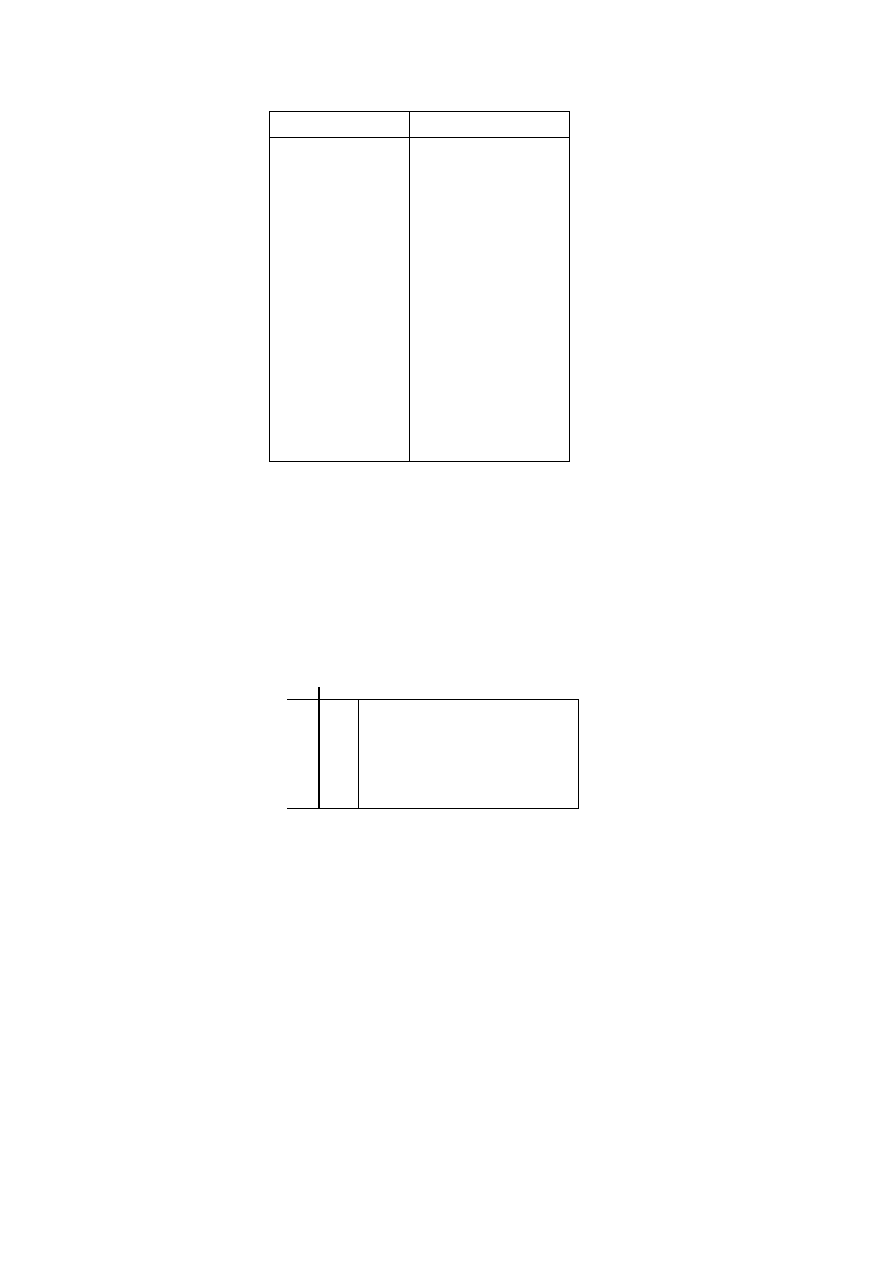ISO/IEC 10918-1 : 1993(E)
Table G.1 ­ EOBn code run length extensions
EOBn code
Run length
EOB0
1
EOB1
2,3
EOB2
4..7
EOB3
8..15
EOB4
16..31
EOB5
32..63
EOB6
64..127
EOB7
128..255
EOB8
256..511
EOB9
512..1 023
EOB10
1 024..2 047
EOB11
2 048..4 095
EOB12
4 096..8 191
EOB13
8 192..16 383
EOB14
16 384..32 767
The end-of-band run structure allows efficient coding of blocks which have only zero coefficients. An EOB run of length
5 means that the current block and the next four blocks have an end-of-band with no intervening non-zero coefficients.
The EOB run length is limited only by the restart interval.
The extension of the code table is illustrated in Figure G.2.
0
1
2
. . .
RRRR
SSSS
13
14
EOB0
EOB1
.
.
.
EOB14
ZRL
0
1
.
.
.
14
15
TISO1580-93/d096
COMPOSITE VALUES
Figure G.2 ­ Two-dimensional value array for Huffman coding
Figure G.2 [D96] = 4.5 cm = 176 %
The EOBn code sequence is defined as follows. Each EOBn code is followed by an extension field similar to the
extension field for the coefficient amplitudes (but with positive numbers only). The number of bits appended to the EOBn
code is the minimum number required to specify the run length.
If an EOB run is greater than 32 767, it is coded as a sequence of EOB runs of length 32 767 followed by a final EOB run
sufficient to complete the run.
At the beginning of each restart interval the EOB run count, EOBRUN, is set to zero. At the end of each restart interval
any remaining EOB run is coded.
The Huffman encoding procedure for AC coefficients in spectral selection and in the first scan of successive
approximation is illustrated in Figures G.3, G.4, G.5, and G.6.
122
CCITT Rec. T.81 (1992 E)